# NCERT Solutions for Class 12 Physics Chapter 15 Communication Systems

In this chapter, we provide NCERT Solutions for Class 12 Physics Chapter 15 Communication Systems for English medium students, Which will very helpful for every student in their exams. Students can download the latest NCERT Solutions for Class 12 Physics Chapter 15 Communication Systems pdf, free NCERT solutions for Class 12 Physics Chapter 15 Communication Systems book pdf download. Now you will get step by step solution to each question.

## NCERT Solutions for Class 12 Physics Chapter 15 Communication Systems

Question 1.
At which of the following frequency/frequencies the communication will not be reliable for a receiver situated beyond horizon:
(a) 10 kHz
(b) 10 MHz
(c) 1 GHz
(d) 1000 GHz
(b) is correct. Here (c) and (d) frequencies have high penetration power so the earth will absorb them. Radiation (a) of 10 kHz will suffer from the problem of size of antenna.

Question 2.
Frequencies in the UHF range normally propagate by means of
(a) ground waves
(b) sky waves
(c) surface waves
(d) space waves.
(d) space waves.

Question 3.
Digital signals (i) do not provide a continuous set of values, (ii) represent values as discrete steps, (Hi) can utilize only binary system, and (iv) can utilize decimal as well as binary system. Which of the following options is true :
(a) Only (i) and (ii).
(b) Only (ii) and (iii).
(c) Only (i), (ii) and (iii), but not (iv).
(d) AH the above (i) to (iv).
(c) is correct because decimal system is concerned with continuous values (i) to (iii).

Question 4.
Is it necessary for a transmitting antenna to be at the same height as that of the receiving antenna for line- of-sight communication ? A TV transmitting antenna is 81 m tall. How much service area can it cover if the receiving antenna is at the ground level?
For line-of-sight communication it is necessary that the transmitting antenna and receiving antenna should be eye to eye but it is not necessary that they should be at the same height.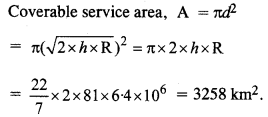Question 5.
A carrier wave of peak voltage 12 V is used to transmit a message signal. What should be the peak voltage of the modulating signal in order to have a modulation index of 75% ?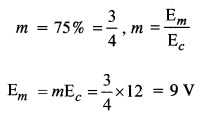Question 6.
A modulation signal is a square wave as shown in figure. The carrier wave is given by
C(t) = 2 sin(8πt) V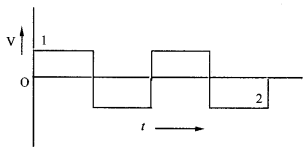(a) Sketch the amplitude modulated waveform.
(b) What is the modulation index ?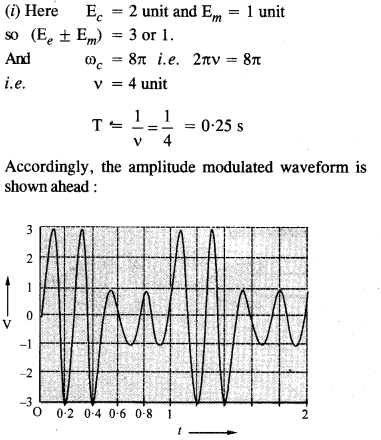Accordingly, the amplitude modulated waveform is shown ahead:

Question 7.
For an amplitude modulated wave, the maximum amplitude is found to be 10 V while the minimum amplitude is found to be 2 V. Determine the modulation index μ. What would be the value of μ if the minimum amplitude is zero V ?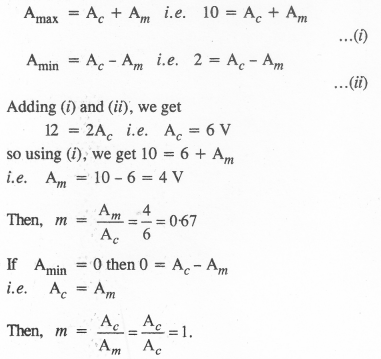Question 8.
Show that if a device is available which can multiply two signals, then it is possible to recover the modulating signal at the receiver station.
Let there be two signals represented by
Ac cos ωct and A0 cos(ωc + ωm)t where Ac is the
amplitude, ωc is the angular frequency of a carrier wave at the receiving end and A0 is the amplitude, (ωc+ ωm) is the angular velocity of the modulated wave.
Multiplying these signals, we getAll Chapter NCERT Solutions For Class 12 Physics

—————————————————————————–

All Subject NCERT Solutions For Class 12

*************************************************

I think you got complete solutions for this chapter. If You have any queries regarding this chapter, please comment on the below section our subject teacher will answer you. We tried our best to give complete solutions so you got good marks in your exam.

If these solutions have helped you, you can also share ncertsolutionsfor.com to your friends.